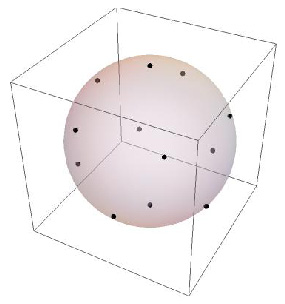#Wolfram Summer School

# Alumni# Emily Payne

Summer School

Class of 2015

## Bio

Emily Payne is a rising 4th year at the University of California, San Diego, currently pursuing a BS in physics (with a specialization in computational physics) and a minor in mathematics. She has worked as an undergraduate research assistant in the fields of philosophy and condensed matter physics.

## Project: Solving the Thomson Problem Using Numerical Minimization Algorithms

The Thomson problem originated as a question posed by the physicist J.J. Thomson after he created his model of the atom (named the plum pudding model). The purpose of the problem is to find the configuration on a unit sphere of a number of electrons, N, that will minimize the total electrostatic potential energy (globally). The electrons repel one other in the magnitude and direction given by Coulomb’s law: F = (q1 q2)/(4π r​2 ϵ​0) This is where the electrostatic potential energy, U(N) = (ΣN-1i=1 ΣNj=i+1)/(rij) is derived from and since this force is proportional to the inverse of the distance between each pair of electrons squared, the stable equilibrium configurations of N electrons are such that the total U(N) of those positions is the global minimum of U(N).

For certain numbers of electrons, there are geometric solutions. The rest must be determined by using numerical minimization algorithms. In my project, I used two of these methods: the NMinimize function in Mathematica, and creating a customized simulated annealing algorithm.## Wolfram Summer School | Champaign, IL, USA | July 3 29–July 22, 2022

APPLY NOW
• 21st Annual Wolfram Summer School
• Bentley University, Waltham, MA, USA
• June 25–July 15, 2023
• Apply now